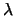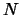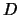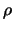Next: Neutral network approach Up: paper2 Previous: Introduction

Model of clusters

The most powerful and widely used methods to calculate the scattering matrix of a fractal cluster are based on the coupled dipoles methods (e.g. the Discrete Dipole Approximation, DDA) and the theory of light scattering by a cluster of spherical particles [2-4]. The latter approach was used by us to obtain the massive of data for neural network training. We construct a model primarily directed at interpretation of the observations of interplanetary dust.

At present the procedure of calculation of the scattering matrix is well developed for both an oriented cluster of spherical particles and for chaotically oriented clusters of such particles . However, the observed dependencies of polarization of light scattered by cometary and interplanetary dust and, in particular, the orientation of the plane of polarization with respect to the scattering plane, allows one to conclude that these dust particles are to be rather chaotically oriented.

Usually to reduce the number of the input parameters one chose the cluster constituent spheres to be identical. For the model of a chaotically oriented cluster of such subparticles, the direct scattering problem has the following input parameters: the size parameter of the constituent spheres(whereis the radius of spheres,the wavelength of incident radiation), the real and imaginary parts of the complex refractive index, the number of particles in the cluster () and parameters describing the cluster structure, which can be characterized by two parameters : the fractal dimensionand a prefactor constant.Next: Neutral network approach Up: paper2 Previous: Introduction
root 2003-04-11